# 划分（你的模式）并修改（您的错误）

[2020-05-14T20:39:29,644][INFO ][index.search.slowlog.fetch.S-OhddFHTc2h6w8NDzPzIw] [instance-0000000000] [kibana_sample_data_flights] took[3.7ms], took_millis, total_hits[416 hits], types[], stats[], search_type[QUERY_THEN_FETCH], total_shards, source[{"query":{"match":{"DestCountry":{"query":"AU","operator":"OR","prefix_length":0,"max_expansions":50,"fuzzy_transpositions":true,"lenient":false,"zero_terms_query":"NONE","auto_generate_synonyms_phrase_query":true,"boost":1.0}}}}], id[],

$%{TIMESTAMP_ISO8601:event.end}$$%{LOGLEVEL:log.level}\s*$$%{DATA:slowlog.type}$\s*$%{DATA:host.name}$\s*$%{DATA:slowlog.index}$\s*$%{DATA:slowlog.shard:int}]took\[%{DATA:slowlog.took}$,\stook_millis$%{DATA:slowlog.took_millis:float}$,\stotal_hits$%{DATA:slowlog.total_hits:int}\shits$\,\stypes$%{DATA:slowlog.types}$,\sstats$%{DATA:slowlog.stats}$,\ssearch_type$%{DATA:slowlog.search_type}$,\stotal_shards$%{DATA:slowlog.total_shards:int}$,\ssource$%{GREEDYDATA:slowlog.source}$,\sid$%{DATA:slowlog.x-opaque-id}$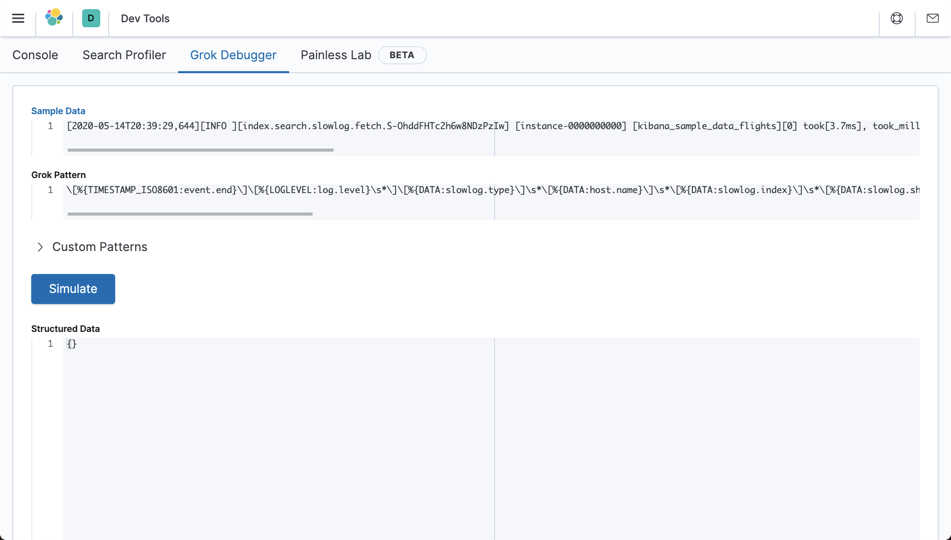%{GREEDYDATA:my_greedy_match}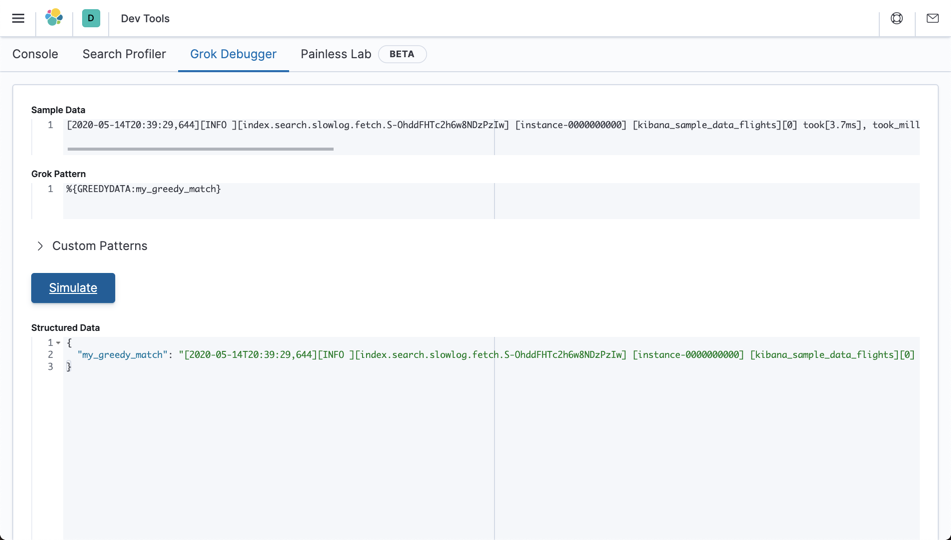$%{TIMESTAMP_ISO8601:event.end}$$%{LOGLEVEL:log.level}\s*$$%{DATA:slowlog.type}$\s*$%{DATA:host.name}$\s*$%{DATA:slowlog.index}$\s*$%{DATA:slowlog.shard:int}]took\[%{DATA:slowlog.took}$,\stook_millis$%{DATA:slowlog.took_millis:float}$,%{GREEDYDATA:my_greedy_match}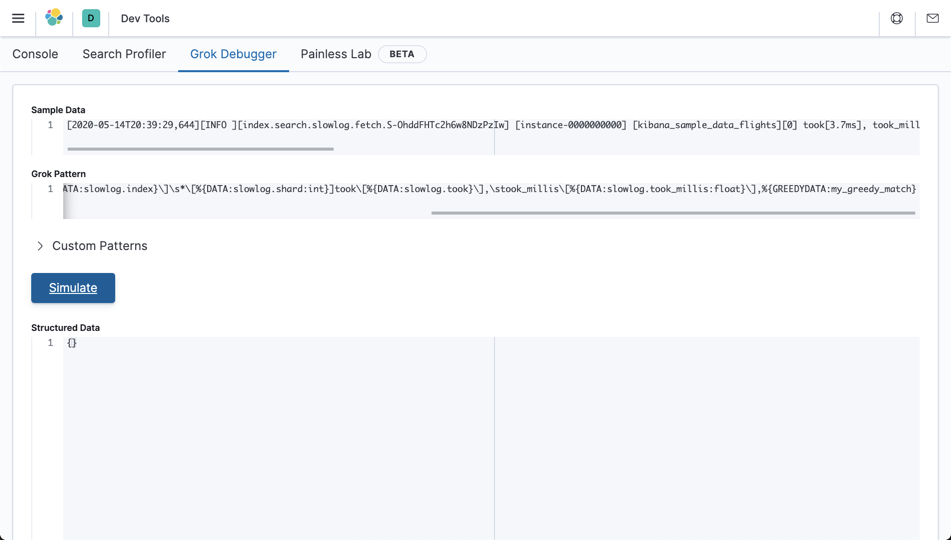$%{TIMESTAMP_ISO8601:event.end}$$%{LOGLEVEL:log.level}\s*$$%{DATA:slowlog.type}$\s*$%{DATA:host.name}$\s*%{GREEDYDATA:my_greedy_match}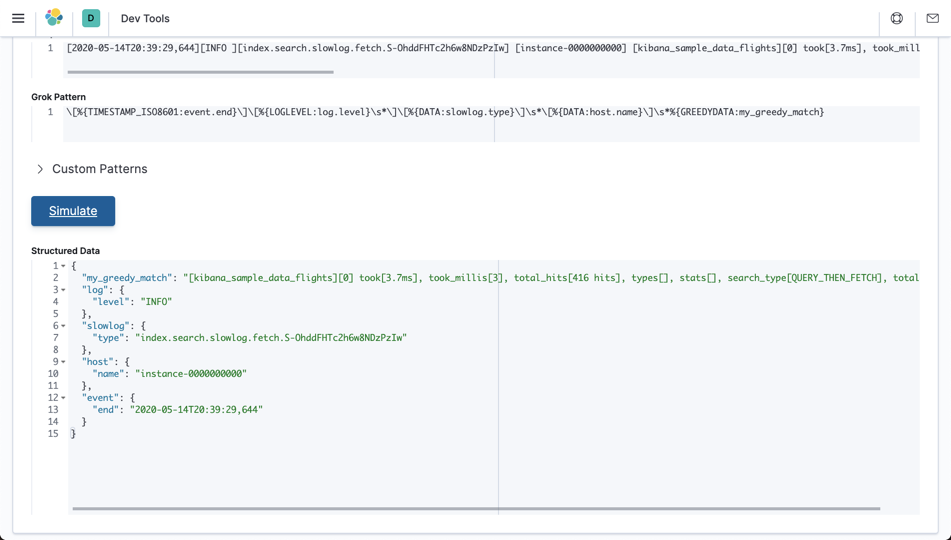$%{TIMESTAMP_ISO8601:event.end}$$%{LOGLEVEL:log.level}\s*$$%{DATA:slowlog.type}$\s*$%{DATA:host.name}$\s*$%{DATA:slowlog.index}$\s*$%{DATA:slowlog.shard:int}]%{GREEDYDATA:my_greedy_match} 在调试器中将如下所示（这是一个匹配项）：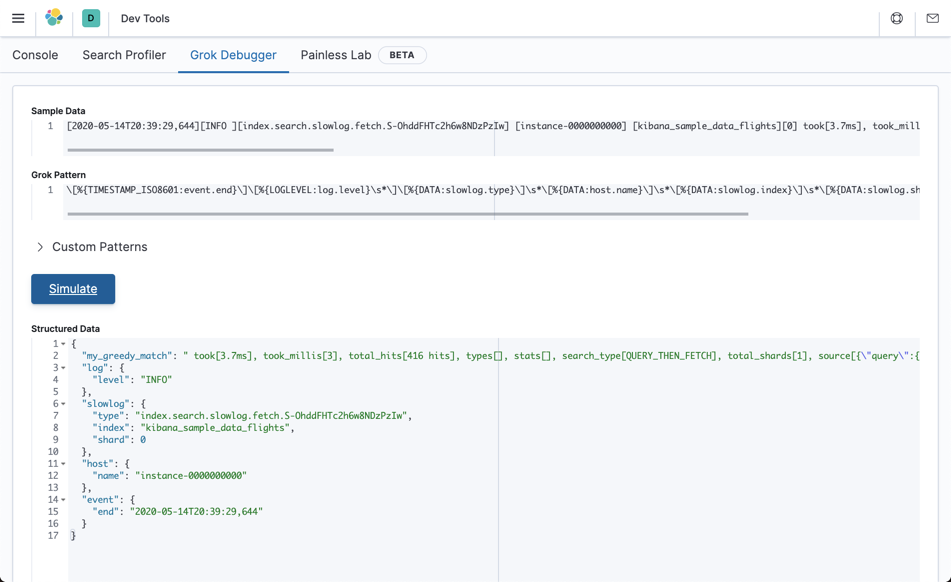因此，我们知道该误差在 grok 模式的八分之三点和中点之间。 让我们尝试添加一些更多的原始 grok 模式，如下所示： \[%{TIMESTAMP_ISO8601:event.end}$$%{LOGLEVEL:log.level}\s*$$%{DATA:slowlog.type}$\s*$%{DATA:host.name}$\s*$%{DATA:slowlog.index}$\s*$%{DATA:slowlog.shard:int}]took%{GREEDYDATA:my_greedy_match} 这将返回一个空响应，如下面的调试器所示： \[%{TIMESTAMP_ISO8601:event.end}$$%{LOGLEVEL:log.level}\s*$$%{DATA:slowlog.type}$\s*$%{DATA:host.name}$\s*$%{DATA:slowlog.index}$\s*$%{DATA:slowlog.shard:int}]took%{GREEDYDATA:my_greedy_match} # 就快到了 提取 slowlog.shard.int 值后出了点问题。 如果我们重新检查我们正在解析的消息，我们将看到被接受的字符串应该在其前面有一个空格字符。 让我们修改 grok 模式，看看在 take 前面指定一个空格时它是否起作用，如下所示： \[%{TIMESTAMP_ISO8601:event.end}$$%{LOGLEVEL:log.level}\s*$$%{DATA:slowlog.type}$\s*$%{DATA:host.name}$\s*$%{DATA:slowlog.index}$\s*$%{DATA:slowlog.shard:int}]\stook%{GREEDYDATA:my_greedy_match} 它起作用，如下所示：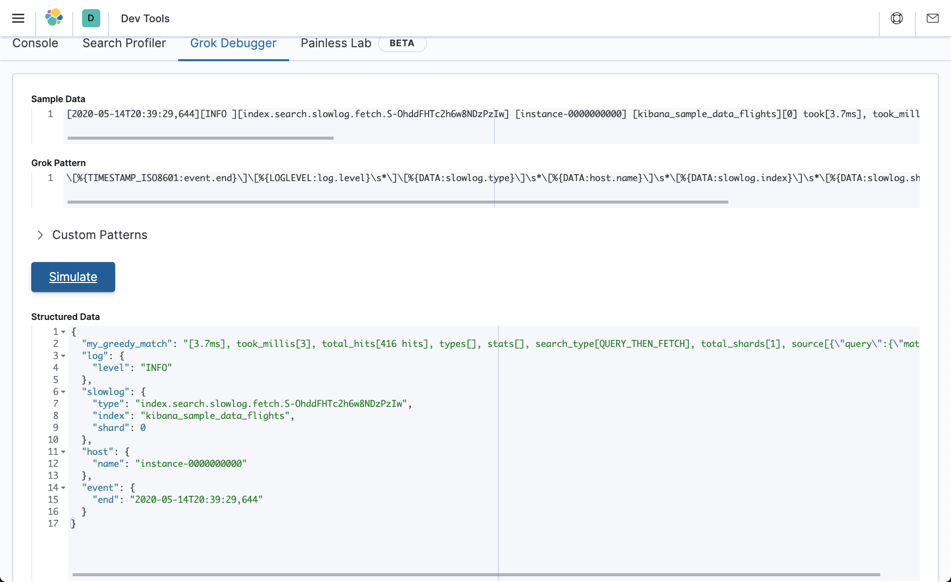但是我们仍然在 my_greedy_match 中存储了一堆数据。 让我们重新添加原始 grok 模式的其余部分，如下所示： \[%{TIMESTAMP_ISO8601:event.end}$$%{LOGLEVEL:log.level}\s*$$%{DATA:slowlog.type}$\s*$%{DATA:host.name}$\s*$%{DATA:slowlog.index}$\s*$%{DATA:slowlog.shard:int}]\stook\[%{DATA:slowlog.took}$,\stook_millis$%{DATA:slowlog.took_millis:float}$,\stotal_hits$%{DATA:slowlog.total_hits:int}\shits$\,\stypes$%{DATA:slowlog.types}$,\sstats$%{DATA:slowlog.stats}$,\ssearch_type$%{DATA:slowlog.search_type}$,\stotal_shards$%{DATA:slowlog.total_shards:int}$,\ssource$%{GREEDYDATA:slowlog.source}$,\sid$%{DATA:slowlog.x-opaque-id}$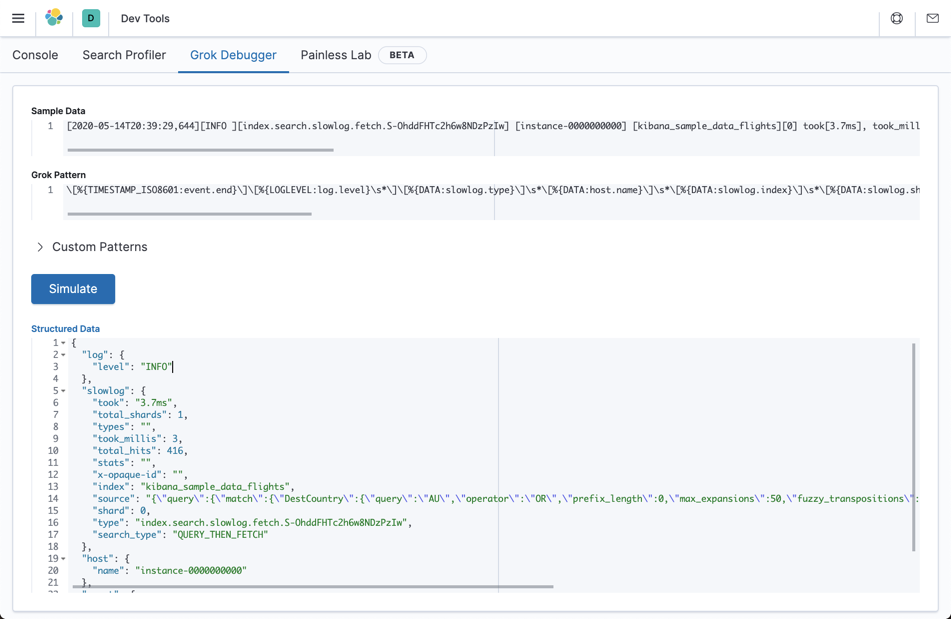grok 模式正在运行！ 现在，我们从以前非结构化的慢日志条目中提取了结构化数据。

# 替代 grok10-282392
07-251993
02-252万+
01-065555
08-137162
08-087228
11-2564
11-2444
11-2348
11-1979